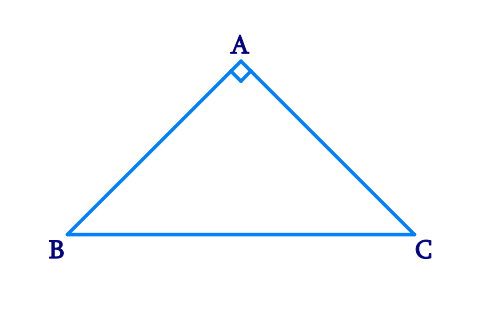# Ex.7.2 Q7 Triangles Solution - NCERT Maths Class 9

Go back to  'Ex.7.2'

## Question

$$ABC$$ is a right-angled triangle in which

$$\angle A = 90^{\circ}$$ and $$AB = AC$$. Find $$\angle B$$ and $$\angle C$$.

Video Solution
Triangles
Ex 7.2 | Question 7

## Text Solution

What is Known?

$$ABC$$ is right-angled triangle and sides $$AB=AC$$

To Find:

Value of $$∠B$$ and $$∠C.$$

Reasoning:

We can use the property angles opposite to equal sides are equal and then by the angle sum property in triangle $$ABC$$ we can find the value of $$∠B$$ and $$∠C.$$

Steps:It is given that

\begin{align} &\quad\, AB=AC\\ &\therefore \angle C=\angle B \\ & \left( \begin{array}{I} \text{Angles opposite to equal sides } \\ \text{of a triangle are also equal}\text{.} \\ \end{array} \right) \\ \end{align}

In $$\Delta ABC,$$

\begin{align} \angle A+\angle B+\angle C&=180^{\circ}\\ (\text{Angle sum property}&\text{ of a triangle}) \\\\ 90^{\circ}+\angle B+\angle C&=180^{\circ} \\ 90^{\circ}+\angle B+\angle B&= 180^{\circ} \\ 2\angle B&= 90^{\circ} \\ \angle B&=45^{\circ} \\ \therefore \angle B=\angle C&=45^{\circ} \\ \end{align}

Video Solution
Triangles
Ex 7.2 | Question 7

Learn from the best math teachers and top your exams

• Live one on one classroom and doubt clearing
• Practice worksheets in and after class for conceptual clarity
• Personalized curriculum to keep up with school